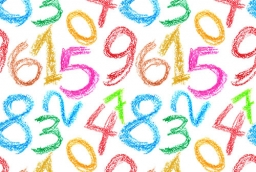# Divisible 9331

The number X is the smallest natural number whose half is divisible by three, a third is divisible by four, a quarter is divisible by eleven, and its half gives a remainder of 5 when divided by seven. Find this number.

X =  528

### Step-by-step explanation:Did you find an error or inaccuracy? Feel free to write us. Thank you!